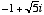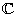index: click on a letter A B C D E F G H I J K L M N O P Q R S T U V W X Y Z A to Z index index: subject areas numbers & symbols sets, logic, proofs geometry algebra trigonometry advanced algebra & pre-calculus calculus advanced topics probability & statistics real world applications multimedia entrieswww.mathwords.com about mathwords website feedback

 Complex Numbers Numbers like 3 – 2i orthat can be written as the sum or difference of a real number and an imaginary number. Complex numbers are indicated by the symbol. Note: All real numbers and all pure imaginary numbers are complex. Sometimes, however, mathematicians use the phrase complex numbers to refer strictly to numbers which have both nonzero real parts and nonzero imaginary parts.See also